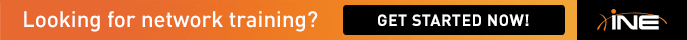# IOS for PPPoE and cell-mode MPLS

Hi Gents

I need an IOS image from 12.2S train that supports both PPPoE and cell-mode MPLS , i'm searching with no luck

i can't find any image supports both features although both are considered SP features

i think these features are supported on T train only but as far as i know all SP core routers are 7200 VXR running 12.2S with different images

can any body confirm other platforms like 3600/3700 can be used in SP core so it could run 12.3T train ?

appreciate your fast reply

Thanks

Hekas

## Comments

• <!>

In the lab you have a mix of 2600, 3600/3700 and 7200:

http://www.cisco.com/web/learning/le3/ccie/sp/lab_equipment.html

The images running on the 7200’s do not support PPPoE (client or
server). During your preparation you should use a combination of 12.2T, 12.3T
and 12.2S.

Check my mini-scenarios for many examples:

http://ccie18473.net/

Regards,

Antonio Soares, CCIE #18473 (R&S/SP)

[email protected]

Antonio Soares, CCIE #18473 (RS/SP/DC)
[email protected]
http://www.ccie18473.net

•

Hi antonio

thanks for your reply but i want to clarify something , you said "The images running on the 7200’s do not support PPPoE (client or server). " do you mean that in the real lab , 7200 images don't support PPPoE functions so always PPPoE server and client are 2600 and 3600/3700 routers ?

did i get you right ?

• <!>

The 7200 routers running IOS 12.2S (SERVICE PROVIDER/SECURE
SHELL 3DES) do not support PPPoE
J

Regards,

Antonio Soares, CCIE #18473 (R&S/SP)

[email protected]

Antonio Soares, CCIE #18473 (RS/SP/DC)
[email protected]
http://www.ccie18473.net

• Thanks antonio for this info -:) , that will help me greatly in my preparation

based in your experience , is there any recommended setup to practice PPPoE and cell-mode on ?

especially  certain IOS images that have bugs like those of the exam

• <!>

See in attach the vrack i used in my preparation.

And you have PPPoE and Cell-Mode scenarios in IESP-VOL2.
Something I highly recommend is the IESP-VOL2 dynamips edition.

Regards,

Antonio Soares, CCIE #18473 (R&S/SP)

[email protected]

Antonio Soares, CCIE #18473 (RS/SP/DC)
[email protected]
http://www.ccie18473.net

• <!>

I forgot that IEOC doesn’t support attachments. Let me try this
way:And the associated .net file:

autostart = false

##################################################################################

[localhost:7200]

[]

image = g:_CCIEEmulatorDynamipsimagesc7200-js.122-11.T.bin

idlepc = 0x606642ac

npe = npe-400

ram = 96

mmap = false

disk0 = 0

##################################################################################

[[Router R1]]

model = 7200

console = 2001

f0/0 = ETHSW 1

s3/0 = R7 s4/0

s3/1 = R7 s4/1

[[Router R2]]

model = 7200

console = 2002

f0/0 = ETHSW 2

s3/0 = R8 s4/0

s3/1 = R8 s4/1

##################################################################################

[localhost:7201]

udp = 11000

[]

image = g:_CCIEEmulatorDynamipsimagesc7200-js.123-8.T11.bin

idlepc = 0x60897efc

npe = npe-400

ram = 128

mmap = false

disk0 = 0

##################################################################################

[[Router R3]]

model = 7200

console = 2003

f0/0 = ETHSW 3

s1/0 = FRSW 3

s1/1 = FRSW 33

s1/2 = R4 s1/2

s1/3 = R4 s1/3

[[Router R4]]

model = 7200

console = 2004

f0/0 = ETHSW 4

S1/0 = FRSW 4

S1/1 = FRSW 44

[[Router R5]]

model = 7200

console = 2005

f0/0 = ETHSW 5

s1/0 = FRSW 5

s1/1 = FRSW 55

s1/2 = R6 s1/2

s1/3 = R6 s1/3

[[Router R6]]

model = 7200

console = 2006

f0/0 = ETHSW 6

s1/0 = FRSW 6

s1/1 = FRSW 66

##################################################################################

[localhost:7202]

udp = 12000

[]

image =
g:_CCIEEmulatorDynamipsimagesc7200-k91p.122-25.S13.bin

ram = 96

npe = npe-400

idlepc = 0x608485e8

mmap = false

disk0 = 0

##################################################################################

[[Router R7]]

model = 7200

console = 2007

f0/0 = ETHSW 7

a1/0 = ATMSW 7

a2/0 = ATMSW 77

a3/0 = BB3 a1/0

[[Router R8]]

model = 7200

console = 2008

f0/0 = ETHSW 8

a1/0 = ATMSW 8

a2/0 = ATMSW 88

a3/0 = BB3 a2/0

[[Router R9]]

model = 7200

console = 2009

f0/0 = ETHSW 9

a1/0 = ATMSW 9

a2/0 = ATMSW 99

a3/0 = BB3 a3/0

##################################################################################

[localhost:7203]

udp = 13000

[]

image = g:_CCIEEmulatorDynamipsimagesc7200-k91p.122-25.S13.bin

ram = 96

npe = npe-400

idlepc = 0x608485e8

mmap = false

disk0 = 0

##################################################################################

[[Router BB1]]

model = 7200

console = 2010

f0/0 = ETHSW 10

[[Router BB2]]

model = 7200

console = 2011

f0/0 = ETHSW 11

[[Router BB3]]

model = 7200

console = 2012

f0/0 = ETHSW 12

##################################################################################

[[ETHSW ETHSW]]

1 = dot1q 1

2 = dot1q 1

3 = dot1q 1

4 = dot1q 1

5 = dot1q 1

6 = dot1q 1

7 = dot1q 1

8 = dot1q 1

9 = dot1q 1

10 = dot1q 1

11 = dot1q 1

12 = dot1q 1

##################################################################################

[[FRSW FRSW]]

3:304 = 4:403

3:305 = 5:503

3:306 = 6:603

33:314 = 44:413

33:315 = 55:513

33:316 = 66:613

4:405 = 5:504

4:406 = 6:604

44:415 = 55:514

44:416 = 66:614

5:506 = 6:605

55:516 = 66:615

##################################################################################

[[ATMSW ATMSW]]

7:0:708 = 8:0:807

7:0:709 = 9:0:907

8:0:809 = 9:0:908

77:1:718 = 88:1:817

77:1:719 = 99:1:917

88:1:819 = 99:1:918

##################################################################################

Regards,

Antonio Soares, CCIE #18473 (R&S/SP)

[email protected]

Antonio Soares, CCIE #18473 (RS/SP/DC)
[email protected]
http://www.ccie18473.net

• Hi Sir,

Really labs are very helpful fo rme ,,, But for PPPOA I am having one question,,,

R1  VCI/VPI 0/101

R2 VCI/VPI 0/201

So when we configure R1 which VPI/VCI Value we need to use.... ??

Waiting for Your reply!

• I didn’t understand your question. In the two PPPoA mini-scenarios, there is one ATM switch providing connectivity:

[[ATMSW ATMSW]]

1:0:102 = 2:0:201

1:0:103 = 3:0:301

2:0:203 = 3:0:302

In other words:

R1---0/102---ATMSW---0/201---R2

R1---0/103---ATMSW---0/301---R3

R2---0/203---ATMSW---0/302R3

Does this answer your question ?

Regards,

Antonio Soares, CCIE #18473 (R&S/SP)
[email protected]

Antonio Soares, CCIE #18473 (RS/SP/DC)
[email protected]
http://www.ccie18473.net

Sign In or Register to comment.